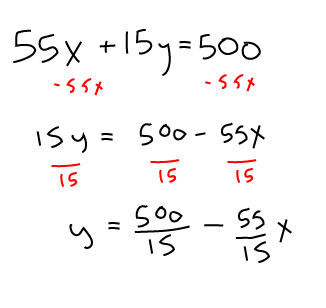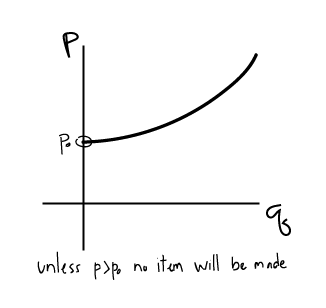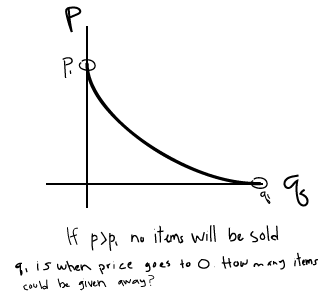# Bloomington Tutors Blog Finite math, K201, calculus, and statistics explained

## The difference between type I and type II errors

Statistics

Statistics is all about trying to make generalizations based on something we can actually see and measure - running an experiment, taking a survey, or considering evidence in a courtroom.

Any time we do this, there is a chance of drawing the wrong conclusion - what we commonly call false positives and false negatives.

In stats classes like K300 and S301, these errors are called by the more confusing names "Type I" and "Type II" errors. In this video Damon gives us an easy way to remember which is which, and why it's important for your exam!

Calculus M119

## 1.4b Budget constraint problem with fixed prices for 2 items

You have a budget of 500 dollars for books ($x$) and CDs ($y$). The total spent on books is $55x$ and the total spent on CDs is $15y$. Write an equation in the form of $y = mx+b$ then solve for $y$.## Supply and demand problems

Supply and demand both relate quantity $q$ of an item to the price $p$.

### Supply curve: Increasing function. $q$ increases as $p$ increases

• The higher the price that sellers can charge for an item, the more quantity they will produce.
• If sellers can NOT sell an item for more money than it costs to manufacture, then they would choose not to manufacture it.### Demand Curve: Decreasing function. $q$ decreases as $p$ increases

• The more an item costs, the less buyers will want to buy it (less quantity will be demanded).
• If price is too high, NO items will be sold.### Equilibrium point: It is assumed that the market settles to an equilibrium point when $S(p) = D(p)$...

Continue Reading...

## Finite Math - Probability with Combinations

Finite M118

This video covers one of the more challenging types of combination and probability problems that are commonly seen on finite exams. We'll need to split the event space into two cases, and compute the number of combinations for each case. Finally, we'll add our two cases together and divide by the total number of combinations in our sample space.

This content was developed with the help of our tutors Grace and Alex, and our design intern Patrick. Thanks everyone!

## Finite Math - Set Theory

Finite M118

Welcome to to the very first video in our finite math series! In this video, we'll talk about the basic concept of sets. Then, we'll use these concepts to frame a simple problem that involves determining how many elements are in a set.

This content was developed with the help of our tutors Grace and Alex, and our design intern Patrick. Thanks everyone!

## GP9 - Text Functions - LEFT, RIGHT, MID, LEN, FIND, and CONCATENATE

Business K201

To be honest, Excel's text functions are kind of awkward and limited. Unlike full-blown programming languages like Python and Javascript, you don't have access to regular expressions or standard string functions such as split, join, and replace. Instead, you have to make due with complicated combinations of Excel's LEFT, RIGHT, MID, LEN, FIND, and CONCATENATE formulas to manipulate text.

In this video, we walk you through a common GP9 string (text) manipulation problem that asks you to use these functions to split, format, and rearrange text into more useful representations.

Download the exercise files

Continue Reading...